# ThreadLevel Parallelism Instructor Adam C Champion Ph D

• Slides: 44
Download presentation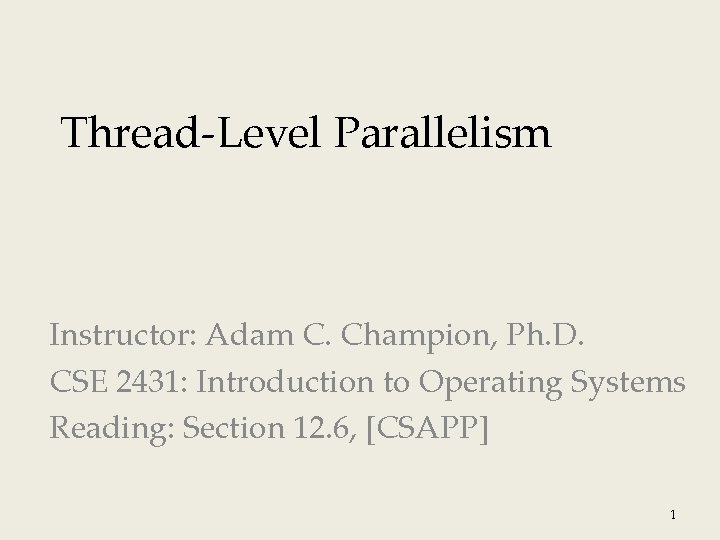Thread-Level Parallelism Instructor: Adam C. Champion, Ph. D. CSE 2431: Introduction to Operating Systems Reading: Section 12. 6, [CSAPP] 1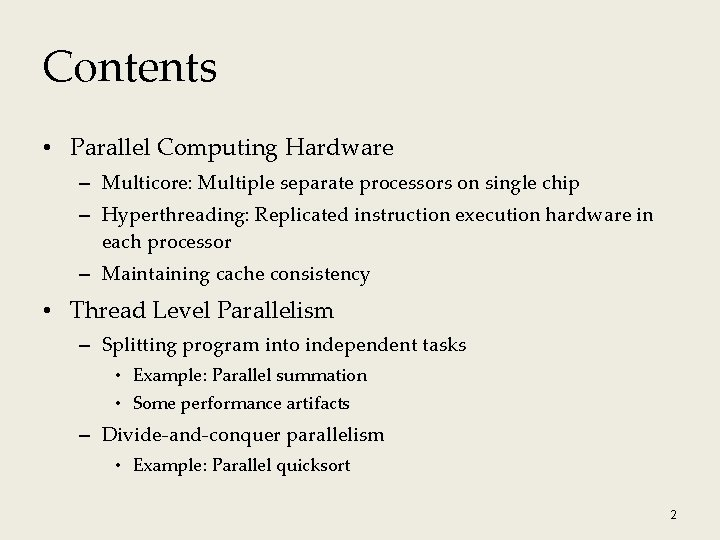Contents • Parallel Computing Hardware – Multicore: Multiple separate processors on single chip – Hyperthreading: Replicated instruction execution hardware in each processor – Maintaining cache consistency • Thread Level Parallelism – Splitting program into independent tasks • Example: Parallel summation • Some performance artifacts – Divide-and-conquer parallelism • Example: Parallel quicksort 2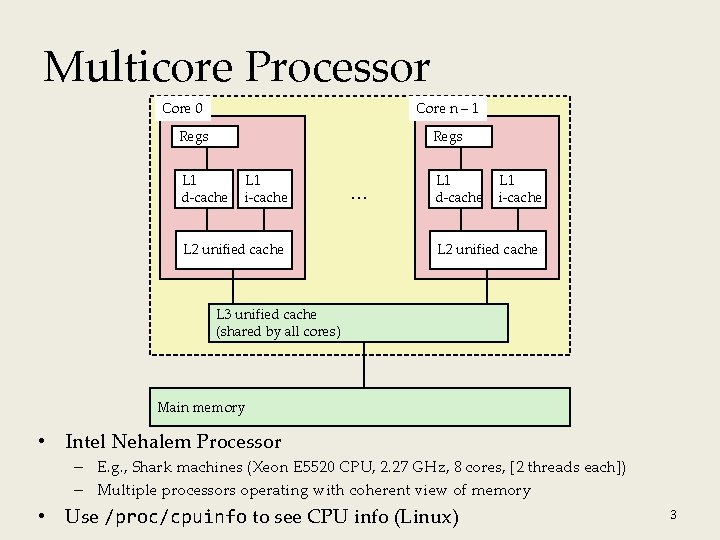Multicore Processor Core 0 Core n – 1 Regs L 1 d-cache L 1 i-cache L 2 unified cache … L 1 d-cache L 1 i-cache L 2 unified cache L 3 unified cache (shared by all cores) Main memory • Intel Nehalem Processor – E. g. , Shark machines (Xeon E 5520 CPU, 2. 27 GHz, 8 cores, [2 threads each]) – Multiple processors operating with coherent view of memory • Use /proc/cpuinfo to see CPU info (Linux) 3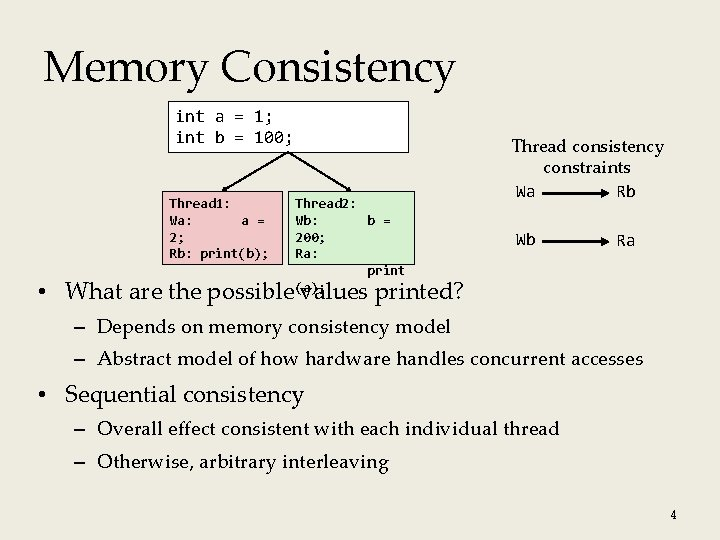Memory Consistency int a = 1; int b = 100; Thread 1: Wa: a = 2; Rb: print(b); Thread 2: Wb: b = 200; Ra: print (a); Thread consistency constraints Wa Rb Wb Ra • What are the possible values printed? – Depends on memory consistency model – Abstract model of how hardware handles concurrent accesses • Sequential consistency – Overall effect consistent with each individual thread – Otherwise, arbitrary interleaving 4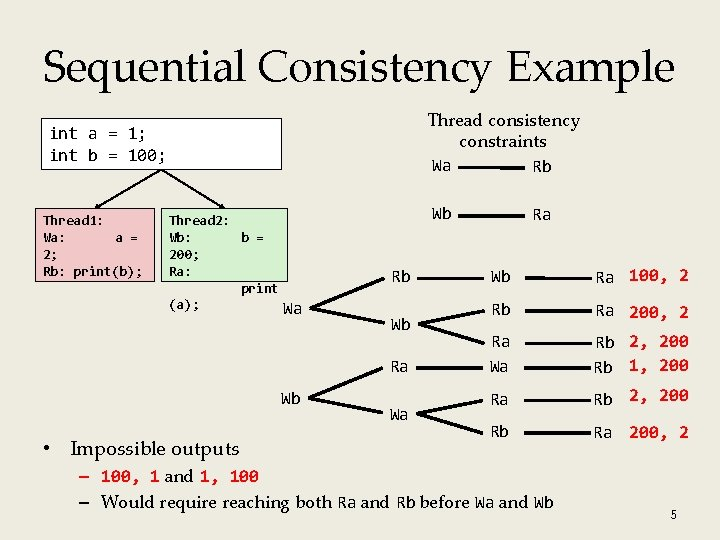Sequential Consistency Example Thread consistency constraints Wa Rb int a = 1; int b = 100; Thread 1: Wa: a = 2; Rb: print(b); Thread 2: Wb: b = 200; Ra: print (a); Wa Wb Rb Wb Ra Wb • Impossible outputs Wa Ra Wb Ra 100, 2 Rb Ra 200, 2 Ra Wa Rb 2, 200 Rb 1, 200 Ra Rb 2, 200 Rb Ra 200, 2 – 100, 1 and 1, 100 – Would require reaching both Ra and Rb before Wa and Wb 5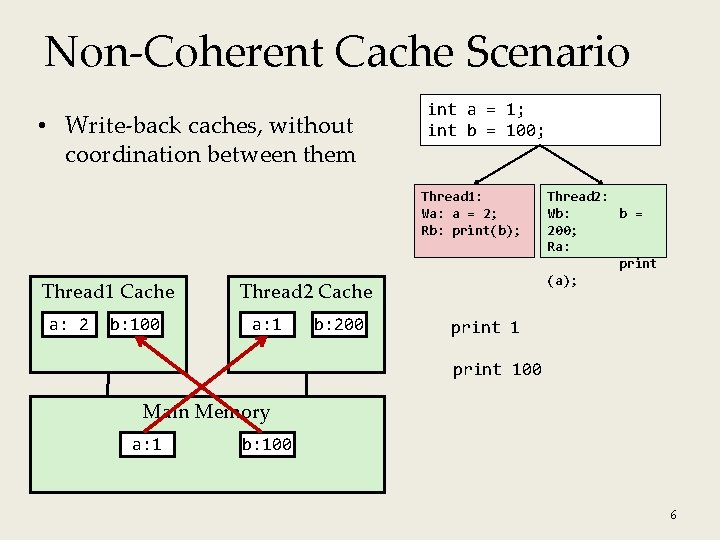Non-Coherent Cache Scenario • Write-back caches, without coordination between them int a = 1; int b = 100; Thread 1: Wa: a = 2; Rb: print(b); Thread 1 Cache a: 2 b: 100 Thread 2 Cache a: 1 b: 200 Thread 2: Wb: b = 200; Ra: print (a); print 100 Main Memory a: 1 b: 100 6Snoopy Caches (1) • Tag each cache block with state Invalid Shared Exclusive Cannot use value Readable copy Writeable copy Thread 1 Cache Thread 2 Cache E a: 2 int a = 1; int b = 100; Thread 1: Wa: a = 2; Rb: print(b); Thread 2: Wb: b = 200; Ra: print (a); E b: 200 Main Memory a: 1 b: 100 7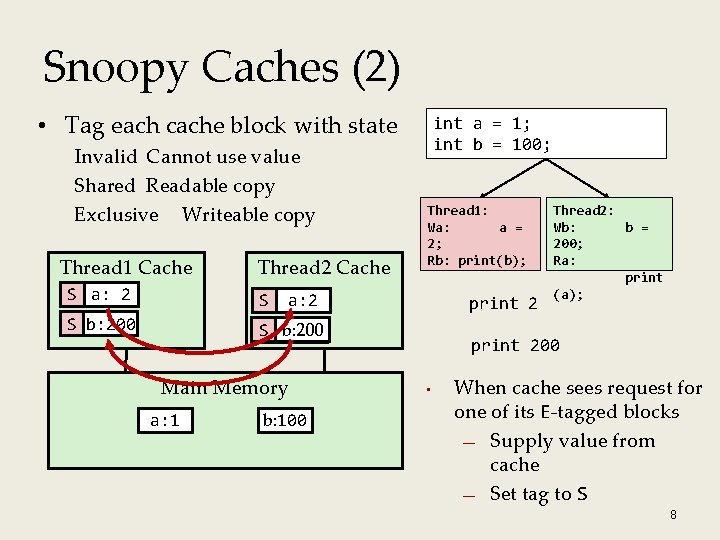Snoopy Caches (2) • Tag each cache block with state Invalid Cannot use value Shared Readable copy Exclusive Writeable copy Thread 1 Cache Thread 2 Cache E a: 22 S a: S S b: 200 E S b: 200 Thread 1: Wa: a = 2; Rb: print(b); a: 2 Main Memory a: 1 int a = 1; int b = 100; b: 100 print 2 Thread 2: Wb: b = 200; Ra: print (a); print 200 • When cache sees request for one of its E-tagged blocks — Supply value from cache — Set tag to S 8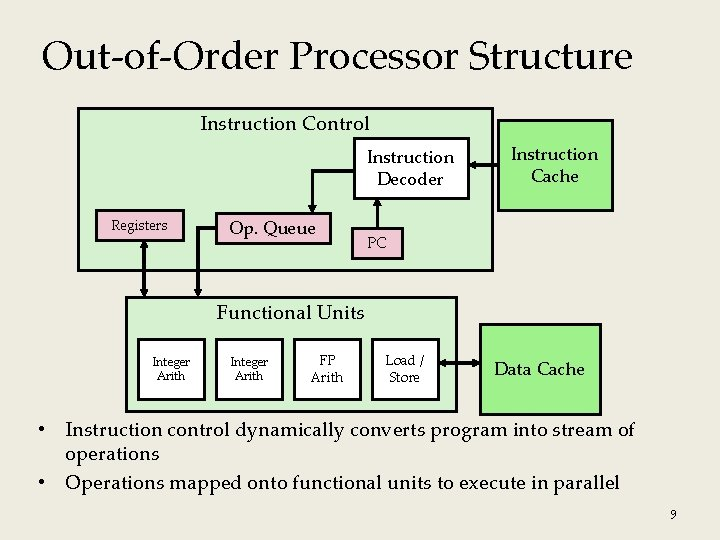Out-of-Order Processor Structure Instruction Control Instruction Decoder Registers Op. Queue Instruction Cache PC Functional Units Integer Arith FP Arith Load / Store Data Cache • Instruction control dynamically converts program into stream of operations • Operations mapped onto functional units to execute in parallel 9Hyperthreading Instruction Control Reg A Instruction Decoder Op. Queue A Reg B Op. Queue B PC A Instruction Cache PC B Functional Units Integer Arith FP Arith Load / Store Data Cache • Replicate enough instruction control to process K instruction streams • K copies of all registers • Share functional units 10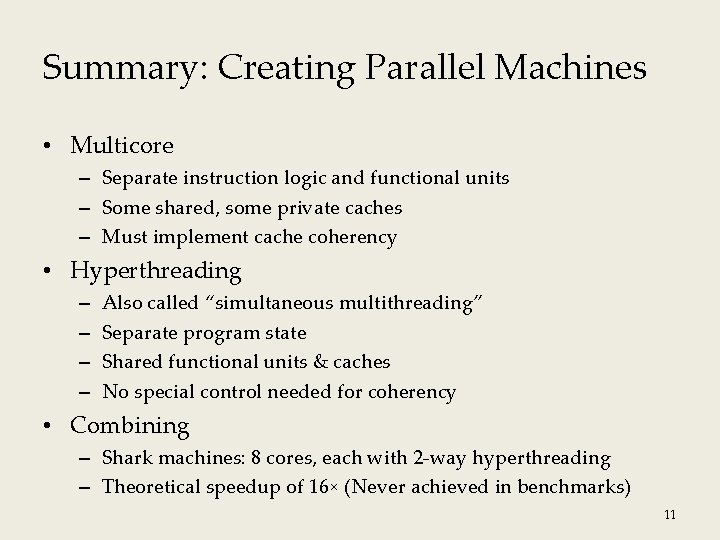Summary: Creating Parallel Machines • Multicore – Separate instruction logic and functional units – Some shared, some private caches – Must implement cache coherency • Hyperthreading – – Also called “simultaneous multithreading” Separate program state Shared functional units & caches No special control needed for coherency • Combining – Shark machines: 8 cores, each with 2 -way hyperthreading – Theoretical speedup of 16× (Never achieved in benchmarks) 11Summation Example • Sum numbers 0, …, N – 1 – Should add up to (N – 1) * N / 2 • Partition into K ranges – N/K values each – Each thread sums one range of numbers – Assume that N is a multiple of K (for simplicity) 12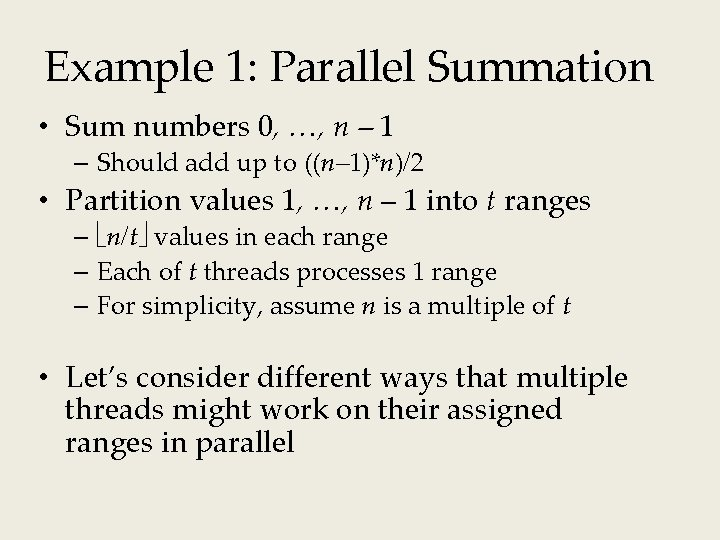Example 1: Parallel Summation • Sum numbers 0, …, n – 1 – Should add up to ((n– 1)*n)/2 • Partition values 1, …, n – 1 into t ranges – n/t values in each range – Each of t threads processes 1 range – For simplicity, assume n is a multiple of t • Let’s consider different ways that multiple threads might work on their assigned ranges in parallelFirst attempt: psum-mutex (1) • Simplest approach: Threads sum into a global variable protected by a semaphore mutex. void *sum_mutex(void *vargp); /* Thread routine */ /* Global shared variables */ long gsum = 0; /* Global sum */ long nelems_per_thread; /* Number of elements to sum */ sem_t mutex; /* Mutex to protect global sum */ int main(int argc, char **argv) { long i, nelems, log_nelems, nthreads, myid[MAXTHREADS]; pthread_t tid[MAXTHREADS]; /* Get input arguments */ nthreads = atoi(argv); log_nelems = atoi(argv); nelems = (1 L << log_nelems); nelems_per_thread = nelems / nthreads; sem_init(&mutex, 0, 1); psum-mutex. c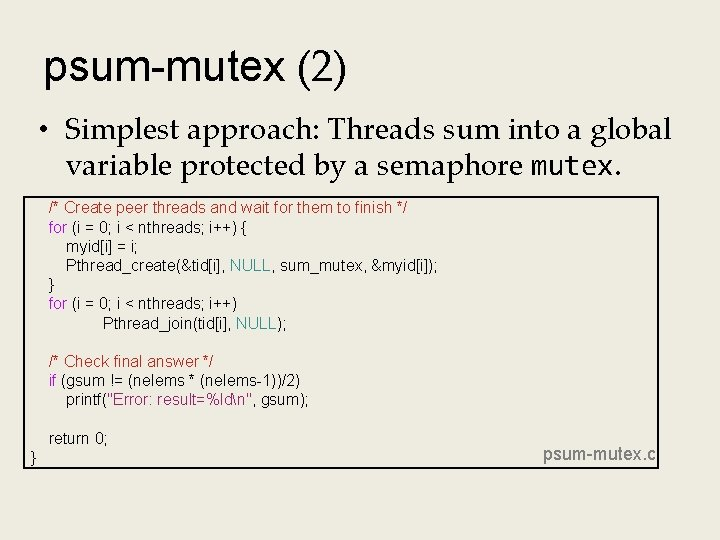psum-mutex (2) • Simplest approach: Threads sum into a global variable protected by a semaphore mutex. /* Create peer threads and wait for them to finish */ for (i = 0; i < nthreads; i++) { myid[i] = i; Pthread_create(&tid[i], NULL, sum_mutex, &myid[i]); } for (i = 0; i < nthreads; i++) Pthread_join(tid[i], NULL); /* Check final answer */ if (gsum != (nelems * (nelems-1))/2) printf("Error: result=%ldn", gsum); return 0; } psum-mutex. c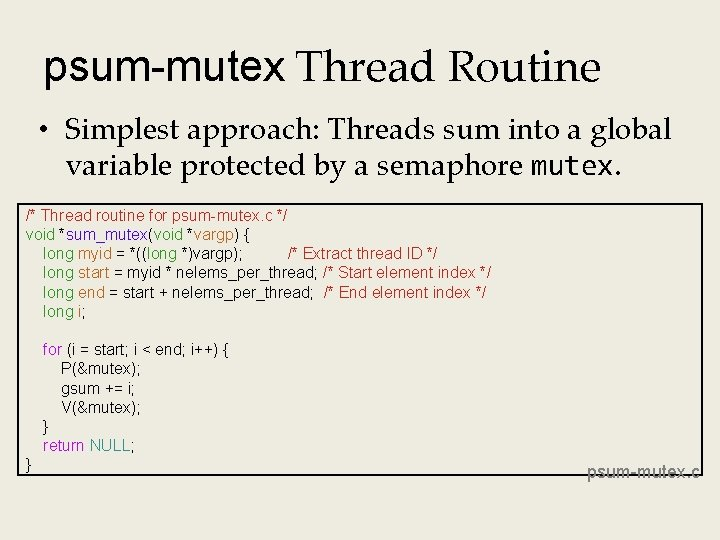psum-mutex Thread Routine • Simplest approach: Threads sum into a global variable protected by a semaphore mutex. /* Thread routine for psum-mutex. c */ void *sum_mutex(void *vargp) { long myid = *((long *)vargp); /* Extract thread ID */ long start = myid * nelems_per_thread; /* Start element index */ long end = start + nelems_per_thread; /* End element index */ long i; for (i = start; i < end; i++) { P(&mutex); gsum += i; V(&mutex); } return NULL; } psum-mutex. cpsum-mutex Performance • Shark machine with 8 cores, n = 231 Threads (Cores) 1 (1) 2 (2) 4 (4) 8 (8) 16 (8) psum-mutex (secs) 51 456 790 536 681 • Nasty surprise: – Single thread is very slow – Gets slower as we use more coresNext Attempt: psum-array • Peer thread i sums into global array element psum[i] • Main waits for threads to finish, then sums elements of psum • Eliminates need for mutex synchronization /* Thread routine for psum-array. c */ void *sum_array(void *vargp) { long myid = *((long *)vargp); /* Extract thread ID */ long start = myid * nelems_per_thread; /* Start element index */ long end = start + nelems_per_thread; /* End element index */ long i; for (i = start; i < end; i++) { psum[myid] += i; } return NULL; } psum-array. c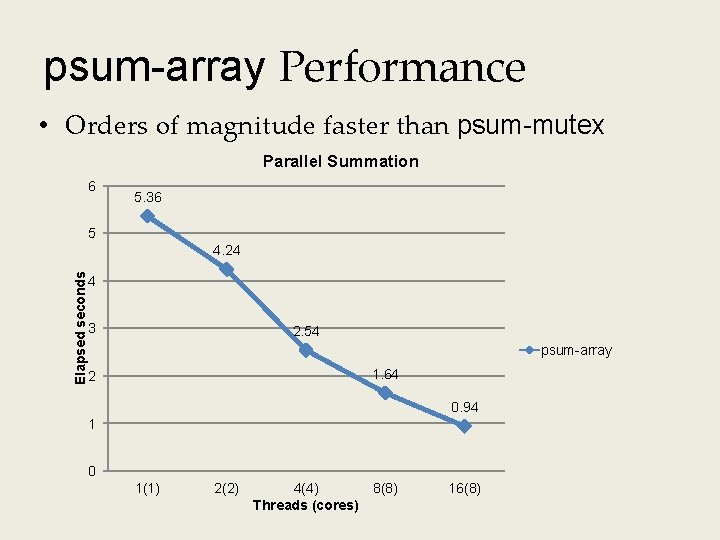psum-array Performance • Orders of magnitude faster than psum-mutex Parallel Summation 6 5. 36 5 Elapsed seconds 4. 24 4 3 2. 54 psum-array 1. 64 2 0. 94 1 0 1(1) 2(2) 4(4) Threads (cores) 8(8) 16(8)Next Attempt: psum-local • Reduce memory references by having peer thread i sum into a local variable (register) /* Thread routine for psum-local. c */ void *sum_local(void *vargp) { long myid = *((long *)vargp); /* Extract thread ID */ long start = myid * nelems_per_thread; /* Start element index */ long end = start + nelems_per_thread; /* End element index */ long i, sum = 0; for (i = start; i < end; i++) { sum += i; } psum[myid] = sum; return NULL; } psum-local. c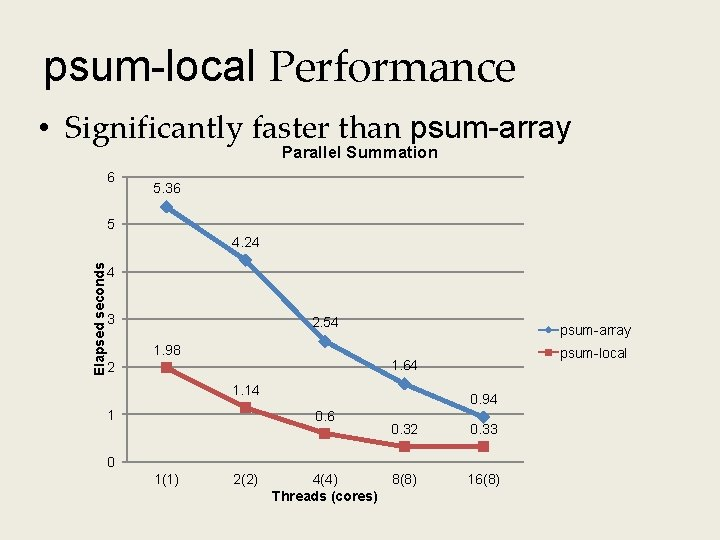psum-local Performance • Significantly faster than psum-array Parallel Summation 6 5. 36 5 Elapsed seconds 4. 24 4 3 2. 54 1. 98 psum-array psum-local 1. 64 2 1. 14 1 0. 94 0. 6 0. 32 0. 33 8(8) 16(8) 0 1(1) 2(2) 4(4) Threads (cores)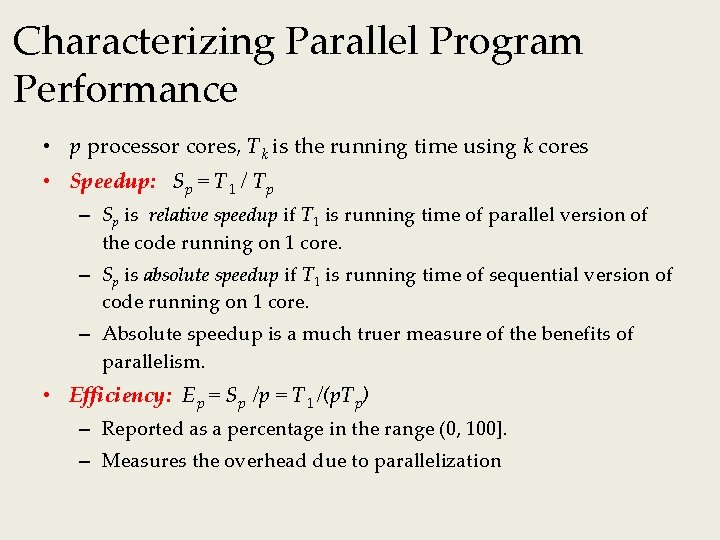Characterizing Parallel Program Performance • p processor cores, Tk is the running time using k cores • Speedup: Sp = T 1 / Tp – Sp is relative speedup if T 1 is running time of parallel version of the code running on 1 core. – Sp is absolute speedup if T 1 is running time of sequential version of code running on 1 core. – Absolute speedup is a much truer measure of the benefits of parallelism. • Efficiency: Ep = Sp /p = T 1 /(p. Tp) – Reported as a percentage in the range (0, 100]. – Measures the overhead due to parallelization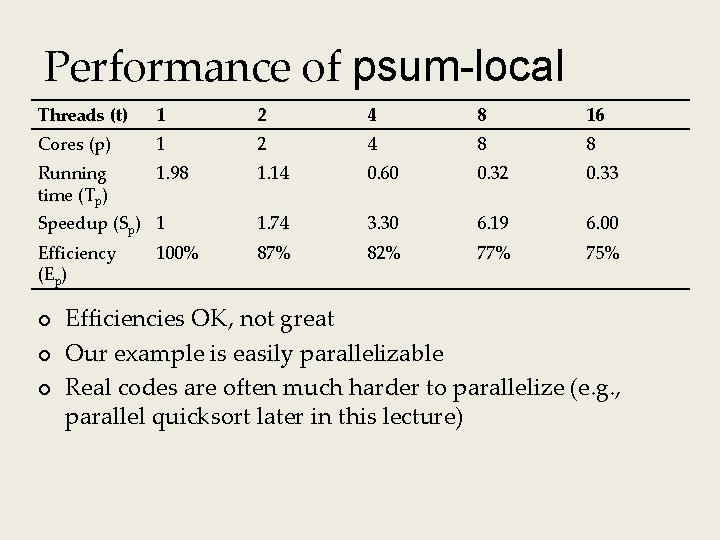Performance of psum-local Threads (t) 1 2 4 8 16 Cores (p) 1 2 4 8 8 Running time (Tp) 1. 98 1. 14 0. 60 0. 32 0. 33 Speedup (Sp) 1 1. 74 3. 30 6. 19 6. 00 Efficiency (Ep) 87% 82% 77% 75% ¢ ¢ ¢ 100% Efficiencies OK, not great Our example is easily parallelizable Real codes are often much harder to parallelize (e. g. , parallel quicksort later in this lecture)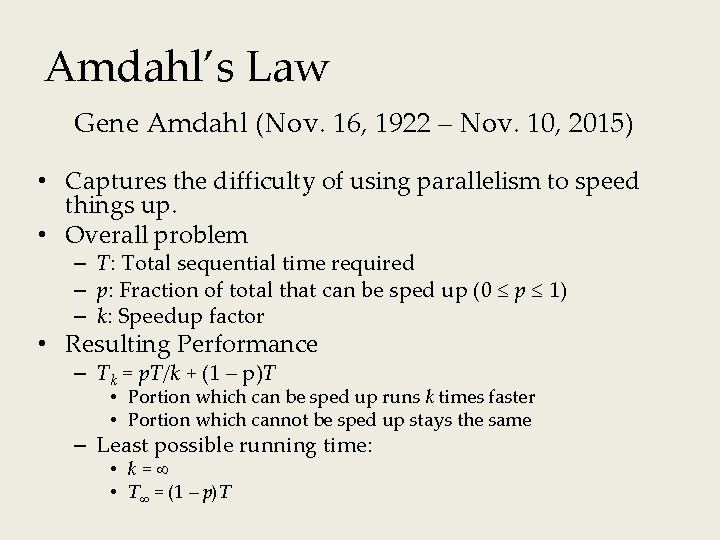Amdahl’s Law Gene Amdahl (Nov. 16, 1922 – Nov. 10, 2015) • Captures the difficulty of using parallelism to speed things up. • Overall problem – T: Total sequential time required – p: Fraction of total that can be sped up (0 p 1) – k: Speedup factor • Resulting Performance – Tk = p. T/k + (1 – p)T • Portion which can be sped up runs k times faster • Portion which cannot be sped up stays the same – Least possible running time: • k= • T = (1 – p)TAmdahl’s Law Example • Overall problem – T = 10 Total time required – p = 0. 9 Fraction of total which can be sped up – k = 9 Speedup factor • Resulting Performance – T 9 = 0. 9 * 10/9 + 0. 1 * 10 = 1. 0 + 1. 0 = 2. 0 – Smallest possible running time: • T = 0. 1 * 10. 0 = 1. 0A More Substantial Example: Sort • Sort set of N random numbers • Multiple possible algorithms – Use parallel version of quicksort • Sequential quicksort of set of values X – Choose “pivot” p from X – Rearrange X into • L: Values p • R: Values p – Recursively sort L to get L – Recursively sort R to get R – Return L : p : R (where : indicates concatenation)Sequential Quicksort Visualized X p L p p 2 L 2 p 2 L R 2 RSequential Quicksort Visualized X L p R p 3 L 3 p 3 R L p R R 3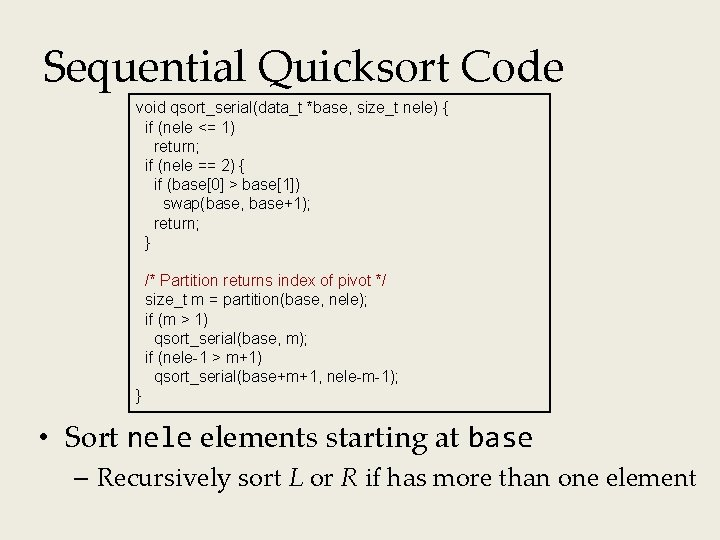Sequential Quicksort Code void qsort_serial(data_t *base, size_t nele) { if (nele <= 1) return; if (nele == 2) { if (base > base) swap(base, base+1); return; } /* Partition returns index of pivot */ size_t m = partition(base, nele); if (m > 1) qsort_serial(base, m); if (nele-1 > m+1) qsort_serial(base+m+1, nele-m-1); } • Sort nele elements starting at base – Recursively sort L or R if has more than one element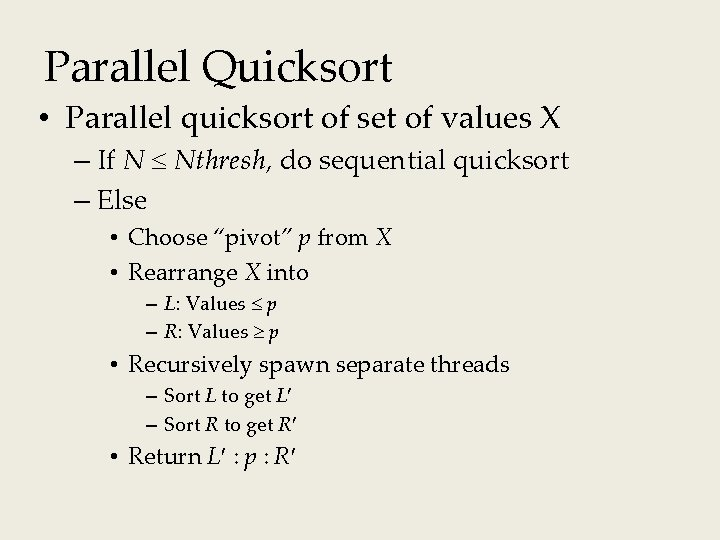Parallel Quicksort • Parallel quicksort of set of values X – If N Nthresh, do sequential quicksort – Else • Choose “pivot” p from X • Rearrange X into – L: Values p – R: Values p • Recursively spawn separate threads – Sort L to get L – Sort R to get R • Return L : p : R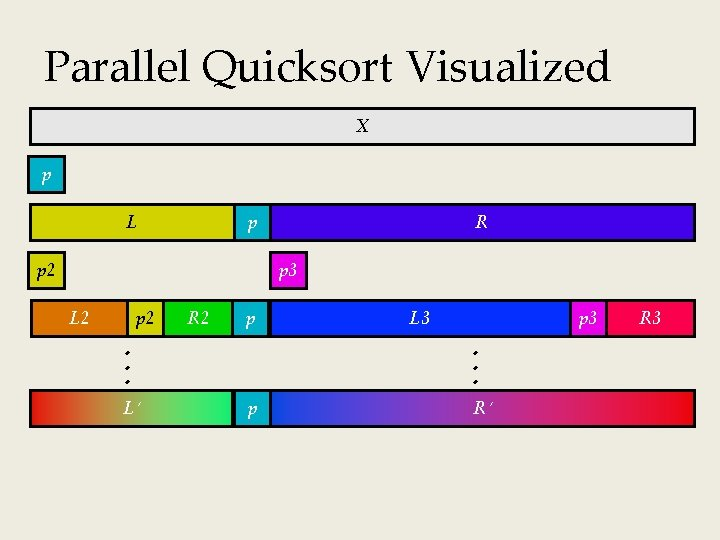Parallel Quicksort Visualized X p L p p 2 R p 3 L 2 p 2 R 2 p L L 3 p 3 p R R 3Thread Structure: Sorting Tasks X Task Threads • Task: Sort subrange of data – Specify as: • base: Starting address • nele: Number of elements in subrange • Run as separate thread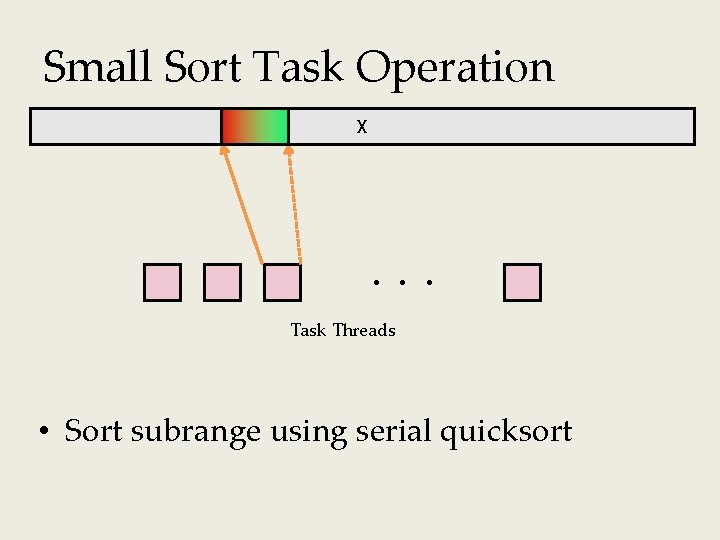Small Sort Task Operation X Task Threads • Sort subrange using serial quicksort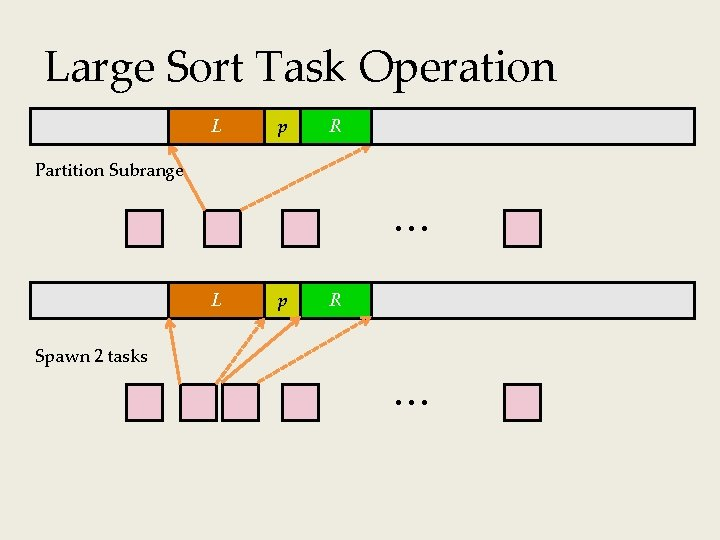Large Sort Task Operation L p R X Partition Subrange L p R X Spawn 2 tasks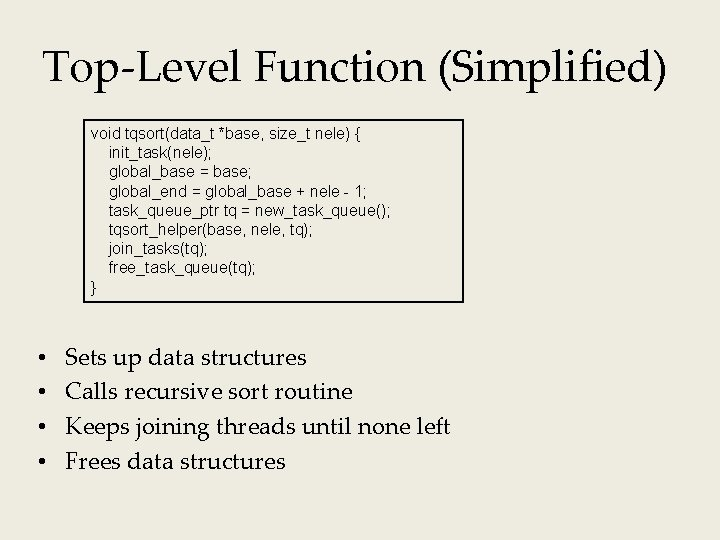Top-Level Function (Simplified) void tqsort(data_t *base, size_t nele) { init_task(nele); global_base = base; global_end = global_base + nele - 1; task_queue_ptr tq = new_task_queue(); tqsort_helper(base, nele, tq); join_tasks(tq); free_task_queue(tq); } • • Sets up data structures Calls recursive sort routine Keeps joining threads until none left Frees data structuresRecursive Sort Routine (Simplified) /* Multi-threaded quicksort */ static void tqsort_helper(data_t *base, size_t nele, task_queue_ptr tq) { if (nele <= nele_max_sort_serial) { /* Use sequential sort */ qsort_serial(base, nele); return; } sort_task_t *t = new_task(base, nele, tq); spawn_task(tq, sort_thread, (void *) t); } • Small partition: Sort serially • Large partition: Spawn new sort task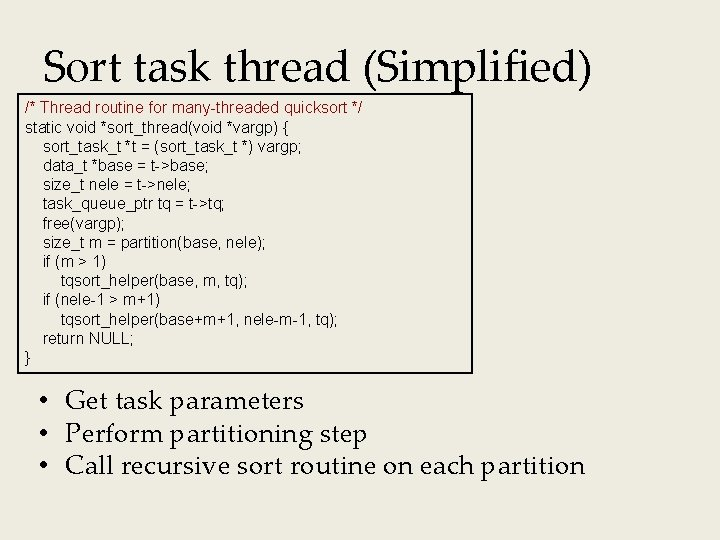Sort task thread (Simplified) /* Thread routine for many-threaded quicksort */ static void *sort_thread(void *vargp) { sort_task_t *t = (sort_task_t *) vargp; data_t *base = t->base; size_t nele = t->nele; task_queue_ptr tq = t->tq; free(vargp); size_t m = partition(base, nele); if (m > 1) tqsort_helper(base, m, tq); if (nele-1 > m+1) tqsort_helper(base+m+1, nele-m-1, tq); return NULL; } • Get task parameters • Perform partitioning step • Call recursive sort routine on each partitionParallel Quicksort Performance (1) • Serial fraction: Fraction of input at which do serial sort • Sort 227 (134, 217, 728) random values • Best speedup = 6. 84×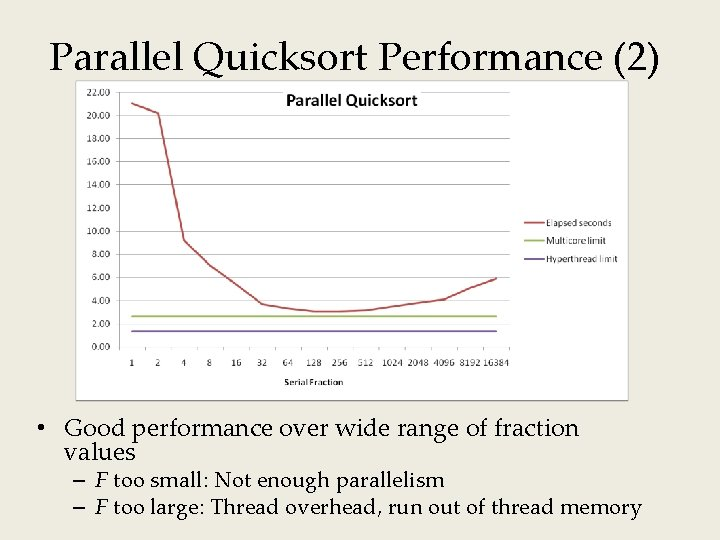Parallel Quicksort Performance (2) • Good performance over wide range of fraction values – F too small: Not enough parallelism – F too large: Thread overhead, run out of thread memoryAmdahl’s Law & Parallel Quicksort • Sequential bottleneck – Top-level partition: No speedup – Second level: 2× speedup – k-th level: 2 k– 1 X speedup • Implications – Good performance for small-scale parallelism – Would need to parallelize partitioning step to get large-scale parallelism • Parallel Sorting by Regular Sampling – H. Shi and J. Schaeffer, J. Parallel & Distributed Computing, 1992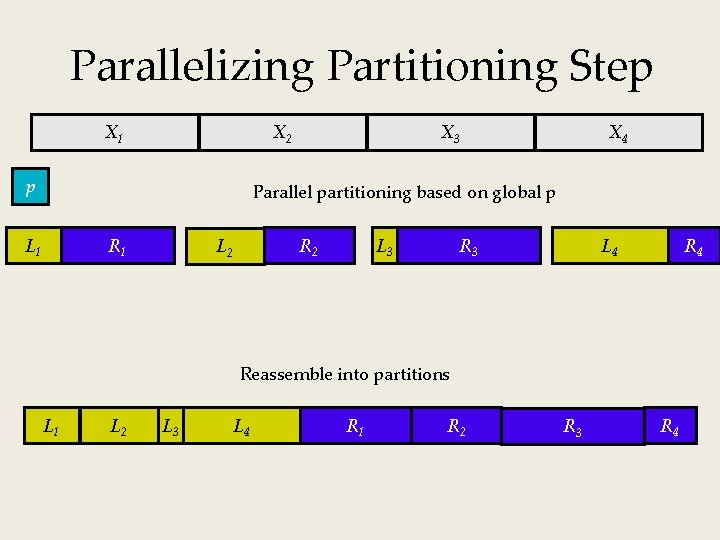Parallelizing Partitioning Step X 1 X 2 p X 3 X 4 Parallel partitioning based on global p R 1 L 1 R 2 L 3 R 4 L 4 Reassemble into partitions L 1 L 2 L 3 L 4 R 1 R 2 R 3 R 4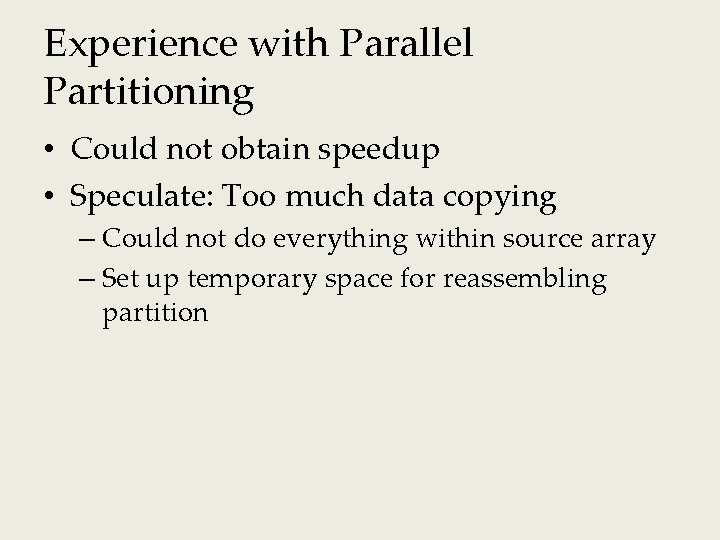Experience with Parallel Partitioning • Could not obtain speedup • Speculate: Too much data copying – Could not do everything within source array – Set up temporary space for reassembling partition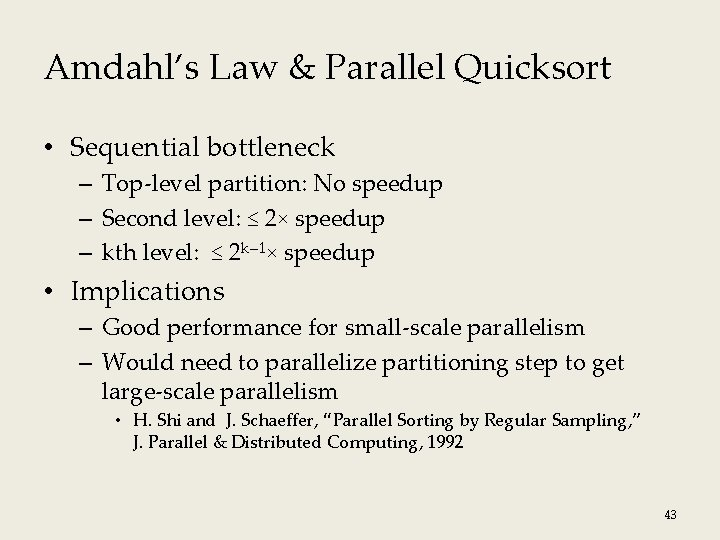Amdahl’s Law & Parallel Quicksort • Sequential bottleneck – Top-level partition: No speedup – Second level: ≤ 2× speedup – kth level: ≤ 2 k– 1× speedup • Implications – Good performance for small-scale parallelism – Would need to parallelize partitioning step to get large-scale parallelism • H. Shi and J. Schaeffer, “Parallel Sorting by Regular Sampling, ” J. Parallel & Distributed Computing, 1992 43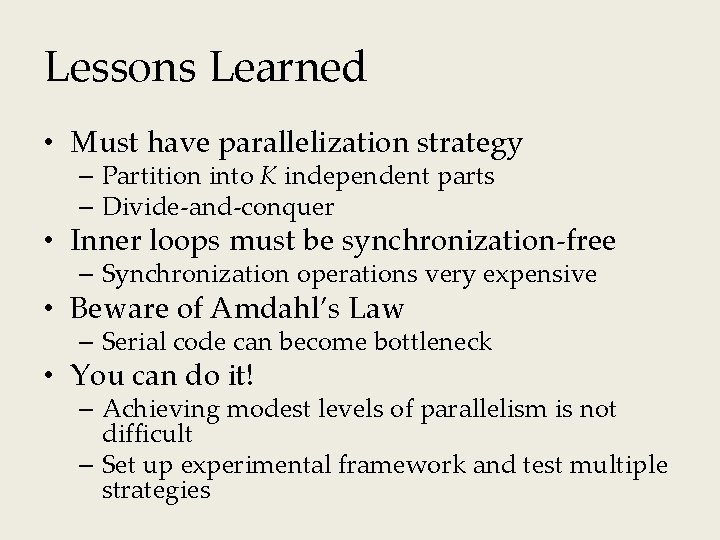Lessons Learned • Must have parallelization strategy – Partition into K independent parts – Divide-and-conquer • Inner loops must be synchronization-free – Synchronization operations very expensive • Beware of Amdahl’s Law – Serial code can become bottleneck • You can do it! – Achieving modest levels of parallelism is not difficult – Set up experimental framework and test multiple strategies